Disclaimer: This is an example of a student written essay.

Any scientific information contained within this essay should not be treated as fact, this content is to be used for educational purposes only and may contain factual inaccuracies or be out of date.

# Enthalpy of Displacement

 ✅ Paper Type: Free Essay ✅ Subject: Chemistry ✅ Wordcount: 2814 words ✅ Published: 29th Jan 2018

• Saran Singh Sound

Aim: To determine the enthalpy change of displacement between zinc powder and copper sulphate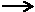Zn (s) + CuSO4 (aq) Cu (s) + ZnSO4 (aq)

Chemicals –

• CuSO­­­4 Solution ( concentration = 0.5 mol.dm3 )
• Zinc (s) Powder

Data Collection –

Table 1: List of apparatus and Least Count and Uncertainties of Measuring Instruments Used

 S. No. Instrument Unit Least Count Uncertainty 1. Butter Paper n/a n/a n/a 2. Cardboard Lid n/a n/a n/a 3. Digital Laboratory Thermometer Celsius 0.1 ºC ±0.1 ºC 4. Digital Weighing Balance Grams 0.001g ±0.001g 5. Measuring Cylinder cm3 1cm3 ±0.5cm3 6. Polystyrene Cup n/a n/a n/a 7. Digital Stopwatch Seconds 0.01s ±0.01s

Qualitative Data

• Time
• Temperature

Table 2.1: Mass of Zinc Powder

 Mass of Zinc (s) /M/g/±0.001g Trial 1 0.244g Trial 2 2.523g Trial 3 2.416g

Table 2.2 : Collected Data

 Time/t/s/±0.01s Temperature/T/°c/±0.1°c Trial 1 Trial 2 Trial 3 30.00 28.4 28.6 60.00 28.4 28.6 90.00 28.4 28.6 120.00 28.4 28.6 150.00 29.1 33.0 180.00 31.2 38.4 210.00 32.9 41.9 240.00 35.0 44.8 270.00 37.5 46.3 300.00 40.0 47.2 330.00 41.6 47.4 360.00 42.5 47.1 390.00 42.5 46.6 420.00 42.3 45.9 450.00 42.1 45.4 480.00 41.6 45.0 510.00 41.0 44.4 540.00 40.5 43.4 570.00 40.0 42.8 600.00 39.4 41.9 630.00 38.7 41.0 660.00 38.0 40.6 690.00 37.4 39.8 720.00 36.6 39.2 750.00 36.0 38.4 780.00 35.5 37.6 810.00 34.7 36.8 840.00 34.0 36.0 870.00 33.2 35.6 900.00 32.6 34.6 930.00 33.7 960.00 32.9 990.00 32.1

Qualitative Observation:

• It was observed that when Zinc (s) powder was added to the CuSO4­ solution it immediately reacted making the solution warmer .The color of the solution at the beginning was greenish in color which then turned colorless after the Zinc (s) powder had reacted. After the reaction was over, it was observed that Copper had precipitated at the bottom of the cup as a result of it being displaced by Zinc (s) powder.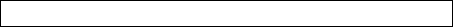Formulas:

Mass = No. of Moles (aq) x Molar Mass (s)And

No. of Moles = Concentration x VolumeAnd

â-²H = Extrapolation Temperature – Initial TemperatureAnd

Enthalpy Change = Mass x Specific Heat Capacity x â-²H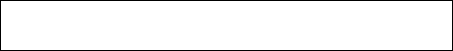And

Molar Enthalpy Change =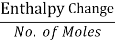And

Mass of water = volume of copper sulphate solution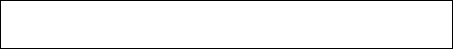And

Percentage Deviation =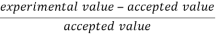x100

Trial 1:

 Volume of CuSO4 (aq) 60.0cm3 Mass of Water 60g Specific Heat Capacity 4.18 J.g‑1.ºC-1 Initial Temperature 28.4 ºC Extrapolation temperature 53.0 ºC Time at which Zinc (s) powder was added 120s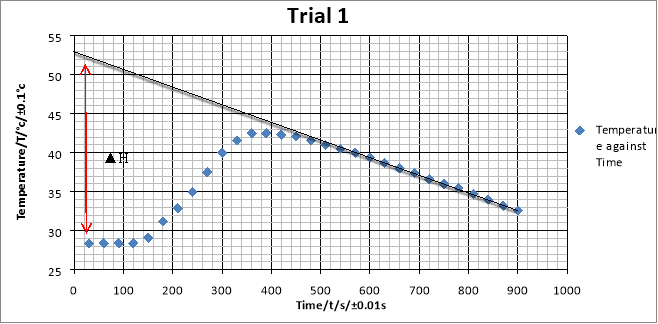The graph is used to estimate the change in temperature of the displacement reaction between CuSO4 solution and Zinc (s) powder. The gradient of the best fit line shows the rate of decrease of temperature.

Calculation:-

No. of Moles of 60.0cm3 CuSO4 (aq) = (60/1000) x 0.5 = 0.03 mol

... Mass of Zinc (s) powder = 0.03 x 65 = 1.961g

However for this experiment, mass of zinc had to be taken in excess. Therefore, Mass of Zinc is greater than actual mass required as seen in Table 2.1

â-²H = 28.4 – 53.0 = -24.60 ºC ±0.01s

Enthalpy Change = 60 x 4.18 x -24.6 = -6169.68 J = -6.16968 kJ

Molar Enthalpy Change =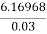= -205.7 kJ.mol-1

As this reaction is exothermic i.e heat is released, enthalpy change is negative.

The literature value of Enthalpy Change for this displacement reaction is -217kJ.mol-1

Percentage Deviation =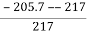x 100 = 5.2% Deviation.

Note: There were two assumptions made during this experiment.

1. The Specific Heat Cpacity of the solution is same as water
2. No Heat is lost to the surrounding

Trial 2:

 Volume of CuSO4 (aq) 70.0cm3 Mass of Water 60g Specific Heat Capacity 4.18 J.g‑1.ºC-1 Initial Temperature 28.6ºC Extrapolation temperature 57.0 ºC Time at which Zinc (s) powder was added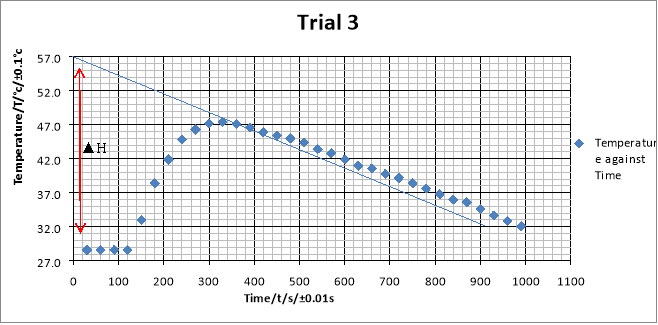The graph is used to estimate the change in temperature of the displacement reaction between CuSO4 solution and Zinc (s) powder. The gradient of the best fit line shows the rate of decrease of temperature.

Calculation:-

No. of Moles of 70.0cm3 CuSO4 (aq) = (70/1000) x 0.5 = 0.035 mol

... Mass of Zinc (s) powder = 0.035 x 65 = 2.275g

However for this experiment, mass of zinc had to be taken in excess. Therefore, Mass of Zinc is greater than actual mass required as seen in Table 2.1

â-²H = 28.6 – 57.0 = -28.40 ºC ±0.01s

Enthalpy Change = 70 x 4.18 x -28.40 = -8309.84 J = -8.30984 kJ

Molar Enthalpy Change =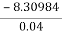= -207.7 kJ.mol-1

As this reaction is exothermic i.e heat is released, enthalpy change is negative.

The literature value of Enthalpy Change for this displacement reaction is -217kJ.mol-1

Percentage Deviation =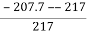x 100 = 4.3% Deviation.

Note: There were two assumptions made during this experiment.

1. The Specific Heat Cpacity of the solution is same as water
2. No Heat is lost to the surrounding

Trial 3:

 Volume of CuSO4 (aq) 70.0cm3 Mass of Water 60g Specific Heat Capacity 4.18 J.g‑1.ºC-1 Initial Temperature 28.6ºC Extrapolation temperature 57.0 ºC Time at which Zinc (s) powder was added 120sThe graph is used to estimate the change in temperature of the displacement reaction between CuSO4 solution and Zinc (s) powder. The gradient of the best fit line shows the rate of decrease of temperature.

Calculation:-

No. of Moles of 70.0cm3 CuSO4 (aq) = (70/1000) x 0.5 = 0.035 mol

... Mass of Zinc (s) powder = 0.035 x 65 = 2.275g

However for this experiment, mass of zinc had to be taken in excess. Therefore, Mass of Zinc is greater than actual mass required as seen in Table 2.1

â-²H = 28.6 – 57.0 = -28.40 ºC ±0.01s

Enthalpy Change = 70 x 4.18 x -28.40 = -8309.84 J = -8.30984 kJ

Molar Enthalpy Change == -207.7 kJ.mol-1

As this reaction is exothermic i.e heat is released, enthalpy change is negative.

The literature value of Enthalpy Change for this displacement reaction is -217kJ.mol-1

Percentage Deviation =x 100 = 4.3% Deviation.

Note: There were two assumptions made during this experiment.

1. The Specific Heat Cpacity of the solution is same as water
2. No Heat is lost to the surrounding

Conclusion:

The displacement reaction between Zinc (s) powder and CuSO4 solution is exothermic as heat is released to its immiediate surroundings. This is supported by the calculations of all the three trials. The Zinc (s) is in powder form which reacts faster with CuSO4 solution as it has a larger surface area. By observing the graph it is found that after reaching the peak temperature, the new solutions temperature starts to decrease which means that its is loosing heat to its surrounding. The line of best fit on the graph shows the temperature change in an ideal situation. However, in reality heat is lost to the surroundings and the specific heat capacity of the solution may not be the same as water. This reaction between Zinc (s) powder and CuSO4­ takes place becaude Zinc (S) powder is more reactive thancopper in CuSO4 solution. Therefore, causing copper to precipitate.

It is also possible that the concentration of the Cuso4 solution was lower , causing less energy to be released then expected.

The temperature change increases as volume of CuSO4 is increased as more Zinc (s) powder is required to react therefore releasing more energy. This is suggested by the calculations for Trial 1, Trial2 and Trial 3.

The percentage deviation of the experimental readings to the literature value is not that high suggesting few errors in the experiment. However, we attributed them to certain and possible errors.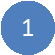View all

## DMCA / Removal Request

If you are the original writer of this essay and no longer wish to have your work published on UKEssays.com then please:

Related Services

Prices from

S\$203

Approximate costs for:

• 1000 words
• 7 day deliveryHumanity University

Dedicated to your worth and value as a human being!

Related Lectures